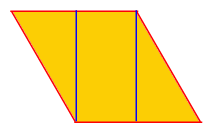Subject: geometry Name: lillian Who are you: Student (Secondary) In a rhombus, each side is 14 in. long. Two of the sides form a 60 degree angle. Find the area of the rhombus. Round your answer to the nearest square inch. Hi Lillian. If you were to cut the end off a rhombus and attach the cut-off triangle to the other side of the rhombus, it would fit perfectly, forming a rectangle, but the area would have to be the same as the original rhombus.Since the area of a rectangle is just the length times the height and you know the length is 14", you just have to calculate the height. Take a closer look at the cut-off piece. It is a right triangle. You know the length of the hypotenuse (14") and one of the angles is 60 degrees, so you can use sine or cosine to determine the height of the rectangle. Hope this helps! Stephen La Rocque.